# Ruled surface

(diff) ← Older revision | Latest revision (diff) | Newer revision → (diff)

A ruled surface in differential geometry is a surface formed by a motion of a straight line. The lines that belongs to this surface are called (rectilinear) generators, and every curve that intersects all the generators is called a directrix. If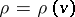is the position vector of the directrix and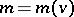is the unit vector of the generator passing through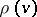, then the position vector of the ruled surface is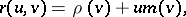whereis the coordinate of a point on the generator. The line element of the ruled surface is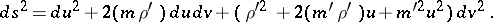A ruled surface is characterized by the fact that its asymptotic net is semi-geodesic. A ruled surface can always be bent in a unique way so that an arbitrary curve on it becomes asymptotic (Beltrami's theorem). Moreover, if a ruled surface that is not developable is bent into another ruled surface, then either their generators coincide or they can both be bent into a quadric on which the net corresponding to the families of generators is asymptotic (Bonnet's theorem).

The set of points of a ruled surface at which the geodesic curvature of the orthogonal trajectories of the generators vanishes is called the line of striction of the ruled surface (or line of contraction, since through each point of it, a point of striction, in the limit there passes the common perpendicular of two infinitely close generators). The coordinate of a point of striction is; on a cylinder the line of striction is undefined, and on a developable surface it is the edge of regression. The limitof the ratio of the shortest distance between two infinitely-close generators of a ruled surface to the angle between them is called the distribution parameter of the ruled surface: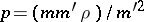; a developable surface is characterized by the fact that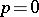for it. The Gaussian curvature of a ruled surface is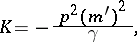where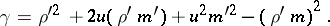The only minimal ruled surface is the helicoid. A ruled surface of revolution is a one-sheet hyperboloid, possibly degenerating to a cylinder, a cone or a plane. If all the generators of a ruled surface are parallel to one plane, then the surface is a Catalan surface.

## Contents

How to Cite This Entry:
Ruled surface. Encyclopedia of Mathematics. URL: http://encyclopediaofmath.org/index.php?title=Ruled_surface&oldid=16245
This article was adapted from an original article by I.Kh. Sabitov (originator), which appeared in Encyclopedia of Mathematics - ISBN 1402006098. See original article